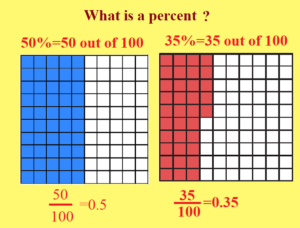December 8, 2023

Percentage questions for class 6

Per means ‘for every’ or ‘out of and cent means ‘hundred’ . Thus percent means ‘ for every hundred’ or ‘out of hundred’. The term percent comes from two Latin words per centum which means ‘out of 100’. Thus  5 percent means 5 out of 100== 5 hundredths. The symbol for percent is

. Example 23% =23 hundredths=# Converting a  Percentage into a Fraction or Decimal:

To convert a given percentage into a fraction or decimal

(i) Remove the sign of percentage  and at the same  time divide by 100.

(ii) Reduce the resulting fraction into its lowest terms  or decimal as required.

Example 1. Express each of the following as fraction.

(a) 40%

Sol.  40 % =

(b) 37.5%

Sol.  37.5 % =

Example 2.  Express each of the following as a decimal.

(a)

Sol.

(b) 7.5 %

S0l. 7.5%=

## Converting a Given Fraction or Decimal into Percentage

To convert a given fraction or decimal into percentage , multiply by 100.

Example 1. Convert

into percentage.

Sol.

Example 2 . Convert  0.08 into a percentage.

Sol.  0.08=(0.08 x 100) % =

### Finding  the Percentage of a Given Number

To find percentage of a number , convert the given percentage to a fraction and  then multiply the fraction by the given number.

Example : Find 6 % of 400.

Sol.  6 % of 400=

#### Convert one Quantity as a Percentage  of the other

Divide the  first quantity by the second one and multiply the result by 100%.

Example 1. Write 20 m as a percentage of 1 km.

Sol.  1 km =1000 m

20 m as a percentage of 1 km=

of a km

Example 2. Express  20 kg  as a percent of  400 kg .

Sol. 20 kg  as a percent of  400 kg  =

##### MCQ on percentage questions for class 6

(i) Convert 40% as a fraction .

(a)

(b)              (c)
(d)

Sol.

Hence  option (a) is correct.

(ii) Convert    into  a percentage.

(a) 25 %          (b) 60 %           (c) 35 %                (d) 75 %

Sol.

Ans.  Option  (d) is correct.

(iii)  Find 8 % of 160 km .

(a) 13 km                (b) 14 km                (c)  12.8 km                    (d) 10 km

Sol.  8 % of 160 km = of 160 km =

km = 12.8 km

Hence option (c) is correct .

(iv) An English test was conducted out of 80 marks. Vani scored 90 %, Tanya scored 85% and Pranoy scored 82.5%. How many marks  did each one score?

(a) 72, 68, 66                 (b)75, 68, 60             (c) 72, 70, 64            (d) 70, 68, 58

Sol. Marks scored by Vani=  90% of 80 =

Marks scored by Tanya = 85% of 80 =

Marks scored by Pranoy = 82.5% of 80 =

Hence option (a) is correct.

(v) Jatin scored  570 marks out of 600. What is the percentage of marks scored?

(a) 80%          (b) 90%          (c) 60%            (d) 95%

Sol. Maximum marks= 600

Marks scored=570

Percentage of marks scored=

. Jatin scored  95% marks.

Option (d) is right.

(vi) What per cent of 85 is 17?

(a) 10%      (b)   20%       (c)  30%       (d)  40%

Sol. Let the required % be . Then

Thus

=20  So the 20% of 85 is 17.  Hence option (b) is correct.

(vii) What percent is 7m 80cm  of 65m ?

(a) 13%        (b) 10 %        (c) 15 %     (d) 12%

Sol.  7m 80cm =780cm

65m=6500cm

Required%= . Option (d) is correct.

(viii)A group of 80 students went on a picnic . 15% of them were girls. How many girls were there in the group? How many were boys?

(a) 12, 68                       (b)15, 30                   (c)    20, 30                (d)   30, 40

Sol. Total number of students =80

Percentage of girls=15%

Number of girls= 15% of 80     =

Number of boys=80-12 =68. Hence option (a) is true.

###### Percentage questions for class 6 PDF